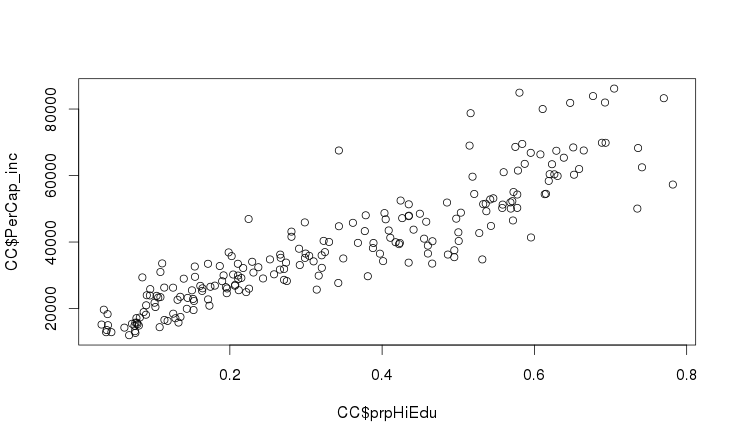# R: Bivariate relation (regression) analysis

December 22, 2014: I have relocated a much more complex analysis of some nonlinear relationships onto a separate subpage.

What is the strength of the relationship between two variables? In statistics, this analytical method is called Simple Linear Regression. Considering what Lennard Davis (1997) argues, I cringe at the idea that we still use Francis Galtons’ term “Regression” for this kind of analysis. In a subtle way, we affirm the idea of eugenics by using Galton’s terminology. Yep, quantitative analysis is saturated with normative–and often racist–ideology. The more we know about its origins, the more we recognize that the idea of quantitative analysis as ‘value-neutral’ is a sick joke. Every technique in human knowledge-production is saturated with ideology. So long as we understand this, we increase the odds that we will know how to use it for good, and not for harm.
I vote for using the term “Relation Analysis” instead. Maybe someday we can retire the jargon used by those who sought to breed the Master Race, and exterminate the rest of us to make room.

In R, the syntax for the Simple Linear Model is lm(y~x).
Before we use this, though, let’s find or calculate some variables that we can relate.

One outcome-variable (“y”) that we can consider is unemployment. We have two variables in our data that can be used to calculate the rate of unemployment in each Tract: not_Working and Work_Age. We could divide not_Working by Total, but then a high number of children (below 16) in the Tract would skew the ratio lower. Ideally, we should also exclude everyone above 65. Can you find the data to do this? It is worth 2 points in your semester grade.

Here is the command for generating an Unemployment variable in dataframe CO:

`CO["unemp"] = CO\$not_Working / CO\$Work_Age`

I also created a variable indicating relative proportion of higher-educated people per tract:

```CO["hiEdu"] = CO\$ED_BA + CO\$ED_transBA
CO["prpHiEdu"] = CO\$hiEdu / CO\$Work_Age```

Again, I used Work_Age as the denominator because it is a little unfair to ask how many children below 16 have a B.A. or higher. Also, after generating this variable in two steps, I realize I could have condensed the creation into one step:

`CO["prpHiEdu"] = (CO\$ED_BA + CO\$ED_transBA) / CO\$Work_Age`

Hopefully, as I show you this “thinking-out-loud” process, it helps reveal how to work with R.

### Graphic analysis of bivariate relationships

Proportion of higher-educated people per Tract with per capita income per tract:

`plot(CO\$prpHiEdu,CO\$PerCap_inc)`The scatterplot shows what we would expect: in tracts with relatively more residents with B.A.s, per capita income is higher. Furthermore, both variables have substantial numbers and a more-or-less “normal” frequency distribution across the whole range of Tracts in the county, so we don’t need to do any transformations to see this relationship. Furthermore, it turns out that the relationship is very strong. How strong is it? How do we describe this relationship numerically?

Stay tuned for our next episode: Simple Linear Modeling!